# Rectangle

Draw a rectangle with the sides a = 4 cm, b = 5 cm. Mark the center of symmetry S and all axes of symmetry. How many axes of symmetry has? Write down.

n =  2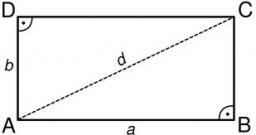Did you find an error or inaccuracy? Feel free to write us. Thank you!#### You need to know the following knowledge to solve this word math problem:

We encourage you to watch this tutorial video on this math problem:

## Related math problems and questions:

• Square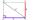Draw a square on the edge of a = 4 cm. Mark the center of symmetry S and all axes of symmetry. How many axes of symmetry does? Write down.
• The sum graphically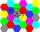Draw a graphically sum of the all sides of 4-gon ABCD.
• TrianglesIvo wants to draw all the triangles whose two sides of which have a length of 4 cm and 9 cm, and the length of the third side is expressed in whole centimeters. How many triangles does he have?
• Draw a trapezoidDraw a trapezoid if given a = 7 cm, b = 4 cm, c = 3.5 cm, diagonal AC = 5cm. Solve as a construction task.
• Rhombus constructionConstruct ABCD rhombus if its diagonal AC=9 cm and side AB = 6 cm. Inscribe a circle in it touching all sides...
• Draw a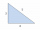Draw a triangle ABC, if you know: alpha = 60° side b = 4 cm side a = 10 cm
• RectanglesHow many different rectangles with sides integers (in mm) have a circumference exactly 1000 cm? (a rectangle with sides of 50cm and 450cm is considered to be the same as a rectangle with sides of 450cm and 50cm)
• Photo frameA square shape photo with a side length of 20 cm is framed by a 4 cm wide bar. Find the external frame size of this photo (the frame is from all sides)
• Three points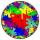Mark three points E, F and G in the plane not lie on one line. a) Draw a line segment FG b) Construct halfline (ray) EG c) Draw a line EF
• Sides od triangleSides of the triangle ABC has length 4 cm, 5 cm and 7 cm. Construct triangle A'B'C' that are similar to triangle ABC which has a circumference of 12 cm.
• Regular octagonDraw the regular octagon ABCDEFGH inscribed with the circle k (S; r = 2.5 cm). Select point S' so that |SS'| = 4.5 cm. Draw S (S '): ABCDEFGH - A'B'C'D'E'F'G'H'.
• Construction of trapezoidConstruct a trapezoid if b = 4cm, c = 7cm, d = 4,5cm, v = 3 cm (Procedure, discussion, sketch, analysis, construction)
• Right triangleDraw a right triangle ABC if |AB| = 5 cm |BC| = 3 cm, |AC| = 4 cm. Draw Thales circle above the hypotenuse of the triangle ABC.
• Square gridSquare grid consists of a square with sides of length 1 cm. Draw in it at least three different patterns such that each had a content of 6 cm2 and circumference 12 cm and that their sides is in square grid.
• Draw it!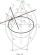Draw two lines c, d that c || d. On line c, mark the points A, B. By point A, a lead perpendicular line to c. By point B, lead perpendicular line to c.
• Trapezoid - constructionConstruct a trapezoid KLMN, where: k = 9 cm, l = 4 cm, m = 5 cm and angle α = 45°5 triangles + 1 square = how many sides in all